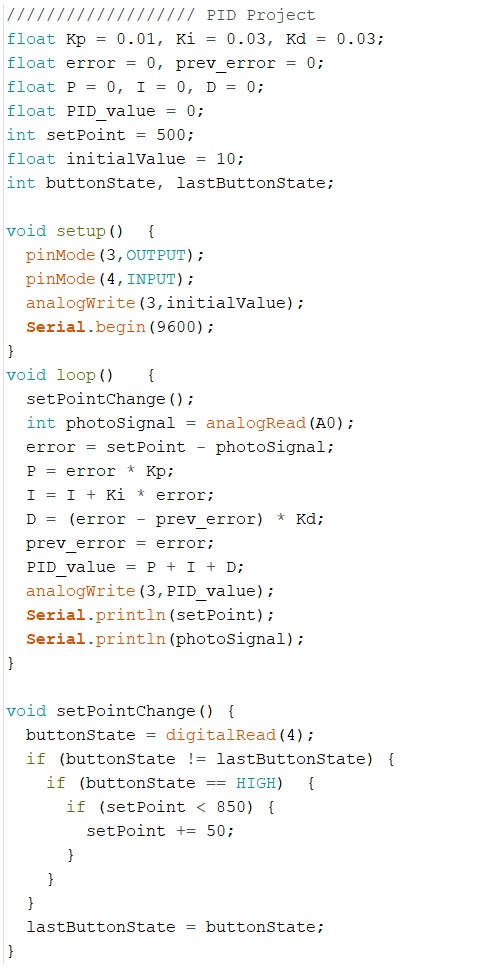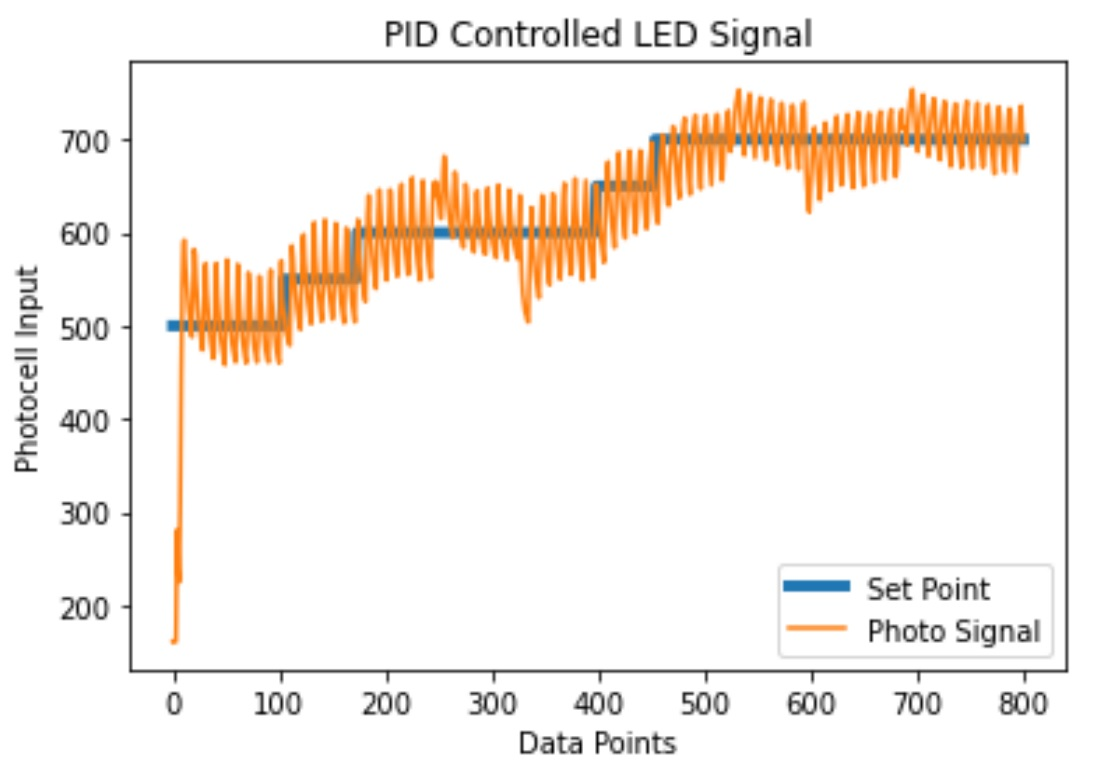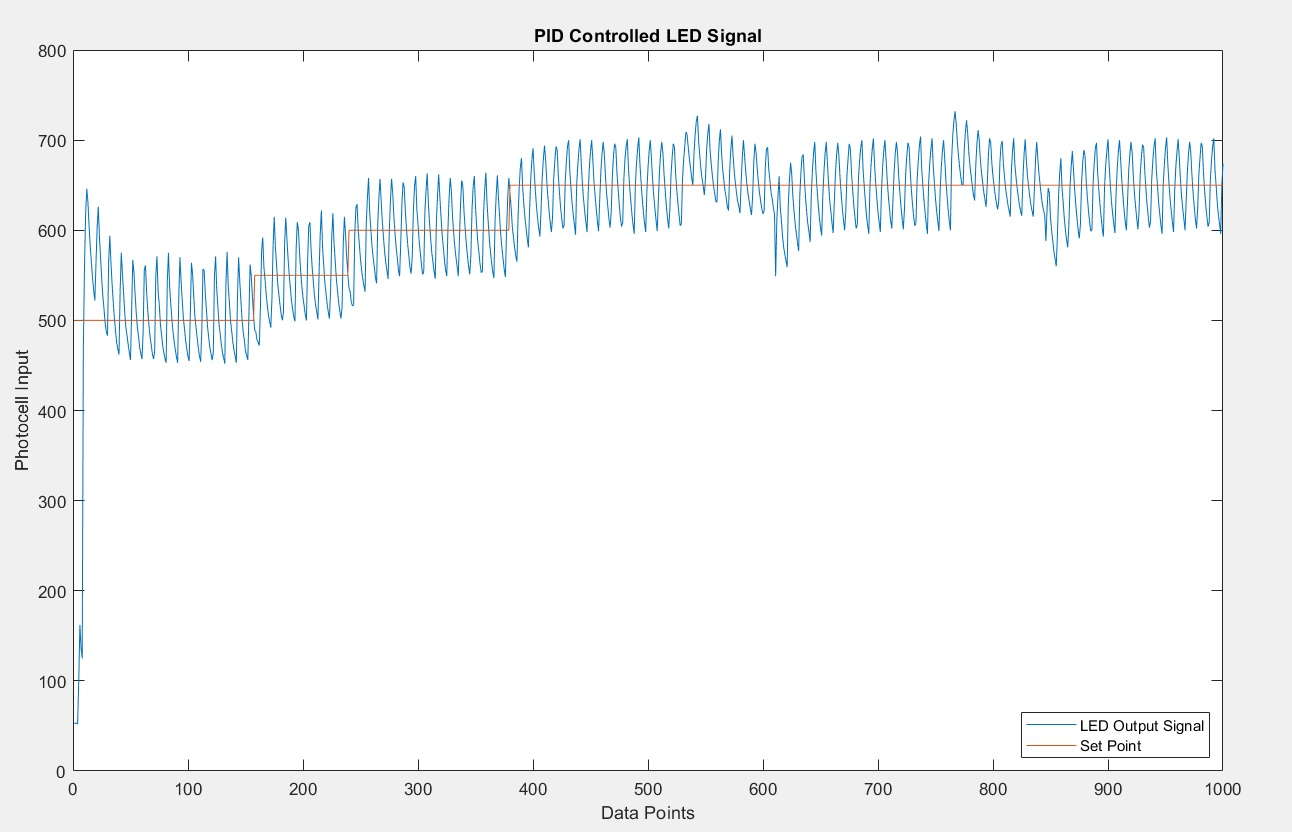CE351 Final Project 2020 Fall
PID Lab continued: Python and Matlab Plotting
Name: Audra Benally
Email: albenally1@fortlewis.edu

1. Introduction
At the end of this semester I had to be quarantined due to potential covid exposure. As a result I was unable to complete the final project for this class. As an alternative project I was assigned further additions to the PID Lab 5. The following are the results: Python and Matlab coding that gather data from the serial port then graph the data with proper labels.

2. The Results

Arduino code:Figure 1. Arduino code that is uploaded to the microcontroller for the Matlab and Python code. I used a method that prints the setPoint and photoSignal on two separate lines; The python and matlab code collect in this specific way too.I have two videos that I recorded using the canvas studio tab. I don't know how to put it in here though. It is in "videos" in this file online.

Python Code that reads data from Serial Port and Plots DataFigure 2. Python graph plotted from data received from the serial port. Both the setpoint tracking and external LED data are recorded in this graph. The LED resets when the code starts, so the first 10 or so data points are taken when the LED is starting up. One issue that happens is the ser.readline() will read two lines which results in a huge spike in the beginning (combined number is in the 100,000 range). It didn't happen very often so I did not think I needed to adjust my code for this phenomenon.

Matlab Code that reads Data from Serial Port and plots on a graph.Figure 3. Matlab data plot from data received from serial port. Both the setpoint tracking and external LED data are recorded in this graph. This data is also taken as the LED starts up: you can see the lower data points in the beginning.